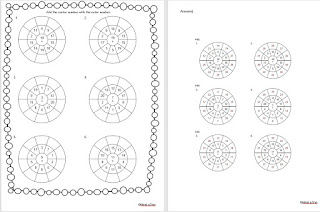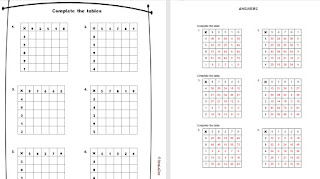Times tables printable to make learning maths fun! Explore times table flash cards, times table games, posters, posters with words, and multiplication tables. Who said learning times tables had to be boring! Let's just play a fun game!

## Sunday, January 28, 2018

Free Short Multiplication Worksheet Year 5 and 6

When two numbers multiplied together and one of them is a single digit, then this short multiplication.
For short multiplication use the smaller number to multiply the larger number one digit at a time work. If the result is ten or more, then the tens amount is carried forward and added to the next column.

﻿﻿﻿Free Printable math Addition worksheet for kids This is a cool maths worksheet. Simple add the numbers to the outer number of the wheel. Just a simple black and white addition worksheet to quickly recap on addition skills.

Mixed Problems Worksheet for Practice

This worksheet has a mixture of math operations: subtraction, division, multiplication and addition. A way to get brains thinking about different operations on one worksheet.
Here is a graphic preview for all of the Mixed Problems Worksheets. Mixed Problems Worksheets to use in the classroom or at home. Our Mixed Problem Worksheets is free to download, easy to use, and very flexible. These Mixed Problems Worksheets are a great resource for children in Kindergarten, 1st Grade, 2nd Grade, 3rd Grade, 4th Grade, and 5th Grade.

####These are great Multiplication Worksheets to test your students understanding of the multiplication times tables. These multiplication times tables worksheets are appropriate for Kindergarten, 1st Grade, 2nd Grade, 3rd Grade, 4th Grade, and 5th Grade.